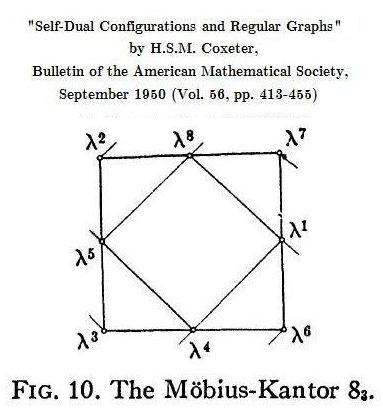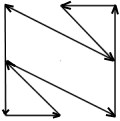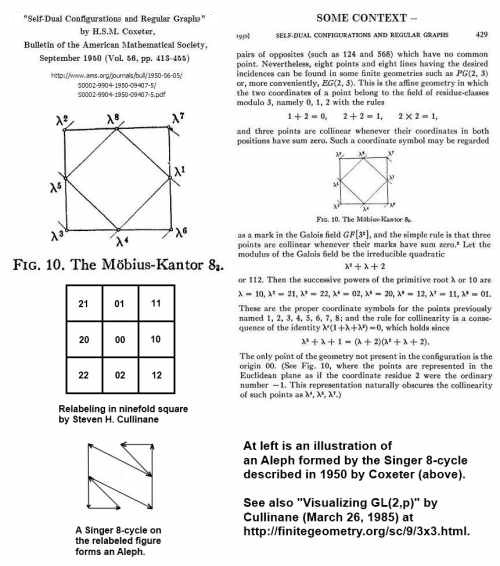# Log24

## Monday, February 20, 2012

### Coxeter and the Relativity Problem

Filed under: General,Geometry — Tags: — m759 @ 12:00 PM

In the Beginning…

"As is well known, the Aleph is the first letter of the Hebrew alphabet."
– Borges, "The Aleph" (1945)

From some 1949 remarks of Weyl—

"The relativity problem is one of central significance throughout geometry and algebra and has been recognized as such by the mathematicians at an early time."

Hermann Weyl, "Relativity Theory as a Stimulus in Mathematical Research," Proceedings of the American Philosophical Society , Vol. 93, No. 7, Theory of Relativity in Contemporary Science: Papers Read at the Celebration of the Seventieth Birthday of Professor Albert Einstein in Princeton, March 19, 1949  (Dec. 30, 1949), pp. 535-541

Weyl in 1946—:

"This is the relativity problem: to fix objectively a class of equivalent coordinatizations and to ascertain the group of transformations S mediating between them."

— Hermann Weyl, The Classical Groups , Princeton University Press, 1946, p. 16

Coxeter in 1950 described the elements of the Galois field GF(9) as powers of a primitive root and as ordered pairs of the field of residue-classes modulo 3—

"… the successive powers of  the primitive root λ or 10 are

λ = 10,  λ2 = 21,  λ3 = 22,  λ4 = 02,
λ5 = 20,  λ6 = 12,  λ7 = 11,  λ8 = 01.

These are the proper coordinate symbols….

(See Fig. 10, where the points are represented in the Euclidean plane as if the coordinate residue 2 were the ordinary number -1. This representation naturally obscures the collinearity of such points as λ4, λ5, λ7.)"Coxeter's Figure 10 yields...The Aleph

The details:Coxeter's phrase "in the Euclidean plane" obscures the noncontinuous nature of the transformations that are automorphisms of the above linear 2-space over GF(3).